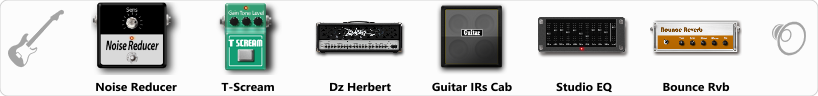# Heavy Rythmic Guitar Sound 2

Discussion in 'ToneLib-GFX presets' started by Rocketes, Oct 10, 2019.

1. Heavy Rythmic Guitar Sound 2

Preset name: Heavy Rythmic Guitar

Effects chain:Effect: "Noise Reducer" (Dynamics / Filter), active - "yes"
"Sens" = 68
"Mode" = Soft

Effect: "T-Scream" (Overdrive / Distortion), active - "yes"
"Drive" = 8
"Tone" = 80
"Level" = 80

Effect: "Dz Herbert" (Amp simulators), active - "yes"
"Gain" = 55
"Bass" = 40
"Middle" = 51
"Treble" = 91
"Presence" = 100
"Master" = 58
"Output" = 50
"Level (dB)" = 3

Effect: "Guitar IRs Cab" (Cabinets), active - "yes"
"Model" = Marshall 1960A (4x12")
"Mic Position" = Middle
"Mic Distance" = Near
"Low Cut (Hz)" = 100
"Hi Cut (kHz)" = 6.0
"Mix" = 100
"Level (dB)" = 0

Effect: "Studio EQ" (Dynamics / Filter), active - "yes"
"31 Hz" = -15
"62 Hz" = -4
"125 Hz" = 1
"250 Hz" = -2
"500 Hz" = -3
"1 kHz" = 1
"2 kHz" = 2
"4 kHz" = 0
"8 kHz" = -4
"16 kHz" = -15
"above 16 kHz" = 0
"Level (dB)" = 0

Effect: "Bounce Rvb" (Reverb), active - "yes"
"Time" = 5.0
"PreDelay" = 0
"LoDamp" = 16
"HiDamp" = 26
"Mix" = 42

Note: You will need to download and install the ToneLib-GFX software to use the preset.

#### Attached Files:

• ###### Heavy_Rythmic_Guitar_Sound_2.tlgfx
File size:
617 bytes
Views:
4,552
vvv, NarFi, Sarcoramphus and 7 others like this.